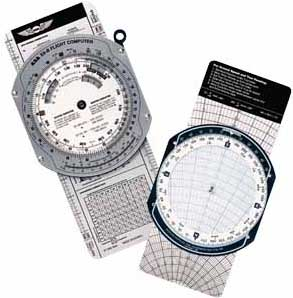# E6B Emulator

This calculator requires the use of Javascript enabled and capable browsers. It is to emulate some of the capabilities of the popular E6B flight computer. Here is the current NOAA aviation weather. For distance between airports, try our Flying Distances Between North American Airports calculator. If the airport you are seeking is not in our table, please send us a quick note and we will add it, assuming we can find longitude and latitude information for it. For distance between specific longitude and latitude points, try our Distances Between Longitude And Latitude Points calculator. Clearing the values will ONLY clear for that calculator so as to retain the other values for other calculations.# E6B Emulator

 Wind Speed Result can be either Knots or MPH based on consistent entry of the same designation. Enter   Ground Speed Enter   True Airspeed Enter   Course Enter   Heading Calculated Wind Speed =
 Wind Direction Result can be either Knots or MPH based on consistent entry of the same designation. Enter   Ground Speed Enter   True Airspeed Enter   Course Enter   Heading Calculated Wind Direction =
 Heading, Ground Speed, And Wind Correction Angle Result can be either Knots or MPH based on consistent entry of the same designation. Enter   Wind Speed Enter   Wind Direction Enter   True Airspeed Enter   Course Calculated   Heading Calculated   Ground Speed Calculated   Wind Correction Angle
 Course, Ground Speed, And Wind Correction Angle Result can be either Knots or MPH based on consistent entry of the same designation. Enter   Wind Speed Enter   Wind Direction Enter   True Airspeed Enter   Heading Calculated   Course Calculated   Ground Speed Calculated   Wind Correction Angle
 Magnetic Variation This calculator is specifically for the Continental USA only Latitude (North Degrees) Enter   - - Longitude (West Degrees) Enter   - - Calculated Variation =
 Magnetic Variation This calculator is specifically for the Alaska region only Latitude (North Degrees) Enter   - - Longitude (West Degrees) Enter   - - Calculated Variation =
 Magnetic Variation This calculator is specifically for the Western Europe region only Latitude (North Degrees) Enter   - - Longitude (West Degrees) Enter   - - Calculated Variation =
 Density Altitude Enter   Pressure Altitude (Ft) Enter   Actual Temperature F° C° Calculated Density Altitude =
 Runway Crosswinds Enter    Runway Compass Heading Enter    Wind (From) Compass Direction Enter    Wind Speed In Knots or MPH Calculated    Crosswind In Knots or MPH From Calculated    Forward Ground Speed In Knots or MPH
 Fahrenheit to Celsius This conversion of temperature is made using the formula Fahrenheit = ((9/5)*Celsius)+32 Enter   °F Calculated Temperature = °C
 Celsius to Fahrenheit This conversion of temperature is made using the formula Celsius = (5/9)*(Fahrenheit-32); Enter   °C Calculated Temperature = °F
 Wind Chill Wind chill cannot be accurately calculated for outside air temperatures (OAT) greater that 50 °F (10 °C) and wind speeds less than 4 MPH (3.5 Knots). Enter   Temperature (OAT) °F °C Enter   Wind MPH Knots Calculated Wind Chill = °F or °C
 Heat Index Determine the heat index from the outside air temperature (OAT) and Relative Humidity. Enter   Temperature (OAT) °F °C Enter   % Relative Humidity Calculated Heat Index = °F or °C
 Dewpoint Calculate the Dewpoint from the outside air temperature (OAT) and Relative Humidity Enter   Temperature (OAT) °F °C Enter   % Relative Humidity Calculated Dewpoint = °F °C
 Relative Humidity Calculate the Relative Humidity from the outside air temperature (OAT) and Dewpoint Enter   Temperature (OAT) °F °C Enter   Dewpoint °F °C Calculated Relative Humidity =  %
 Pressure Conversion Convert Millibars to Inches of Mercury and Inches of Mercury to Millibars Enter   Millibars Inches Mercury Converted Barometric Pressure =
 Miles Per Hour To Knots This conversion of speed (or distance) is made using the formula (Knots (or nautical miles) = MPH (or miles) * 0.86897624) Enter   MPH or Statute Miles Calculated Knots Or Nautical Miles =
 Knots to Miles Per Hour This conversion of speed (or distance) is made using the formula (MPH (or statute miles) = Knots (or nautical miles) * 1.15077945) Enter   Knots or Nautical Miles Calculated MPH or Statute Miles =
 True Airspeed (TAS or KTAS) Result can be either Knots or MPH based on consistent entry of the same designation. The formula is (IAS) + (.02 x MSL / 1000). Enter   Indicated Airspeed Enter   MSL Altitude Calculated True Airpeed =
Version 7.8.9

 Leave us a question or comment on FacebookSearch or Browse Our Site